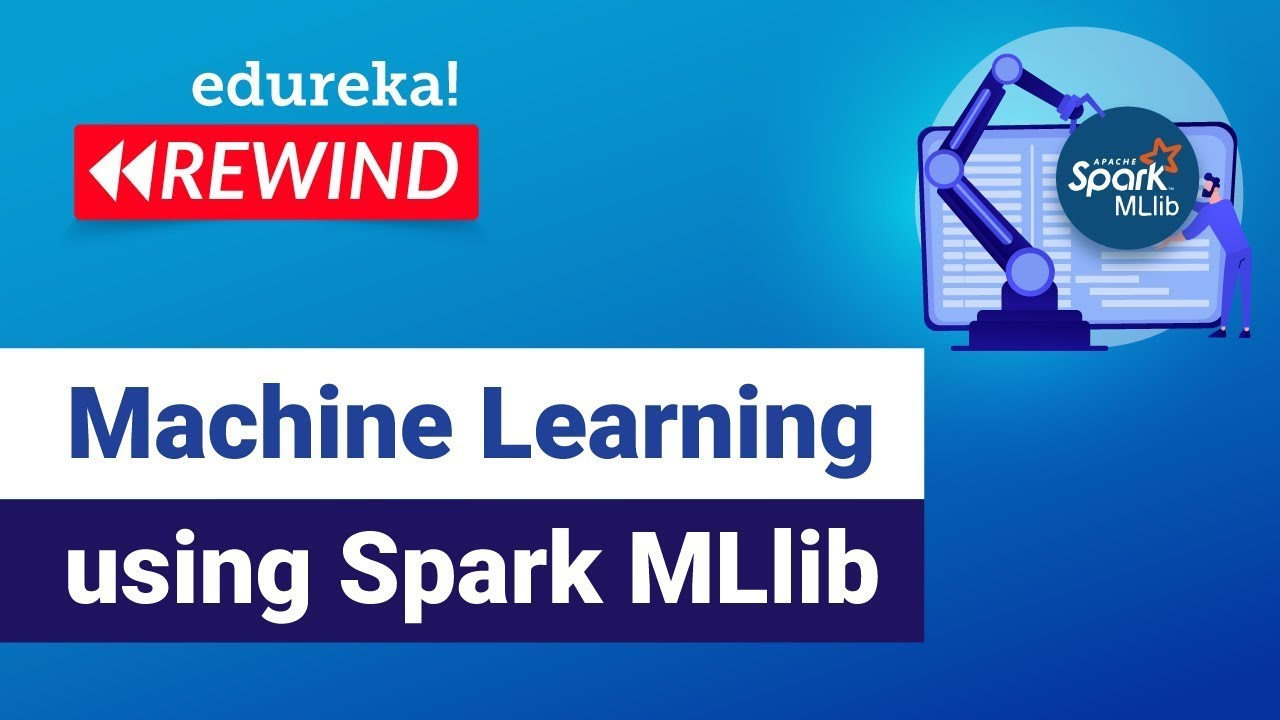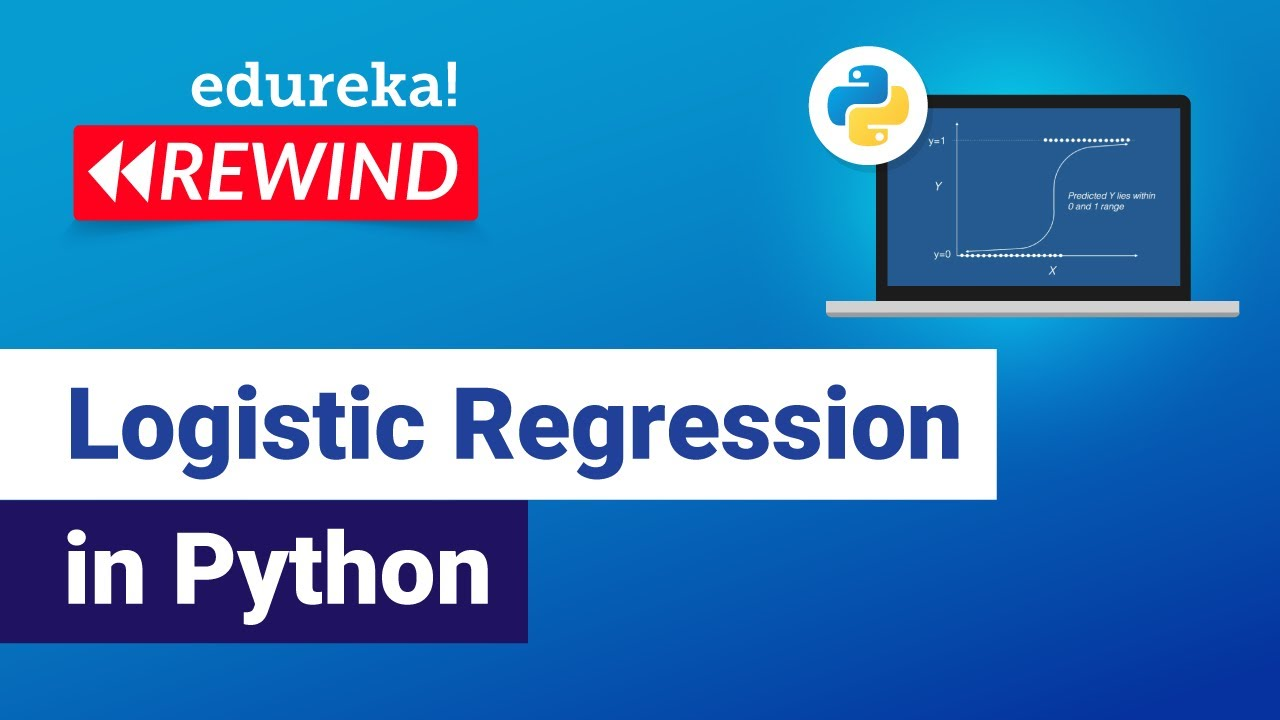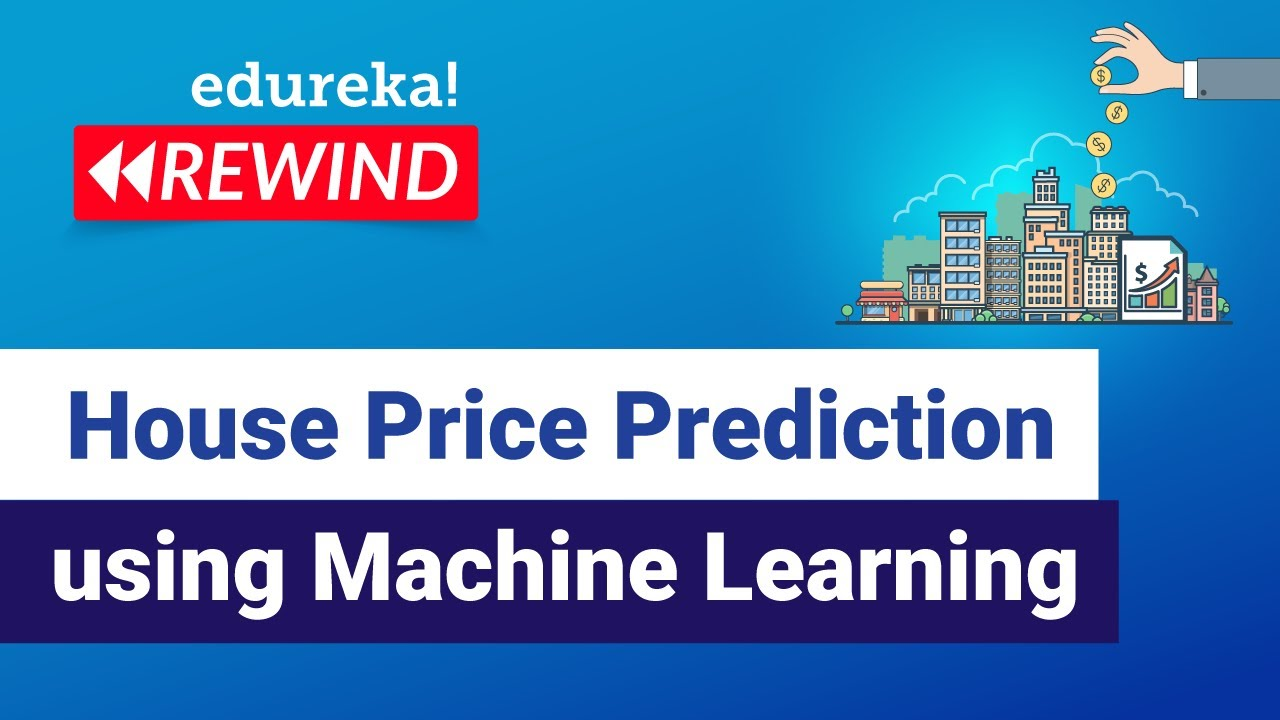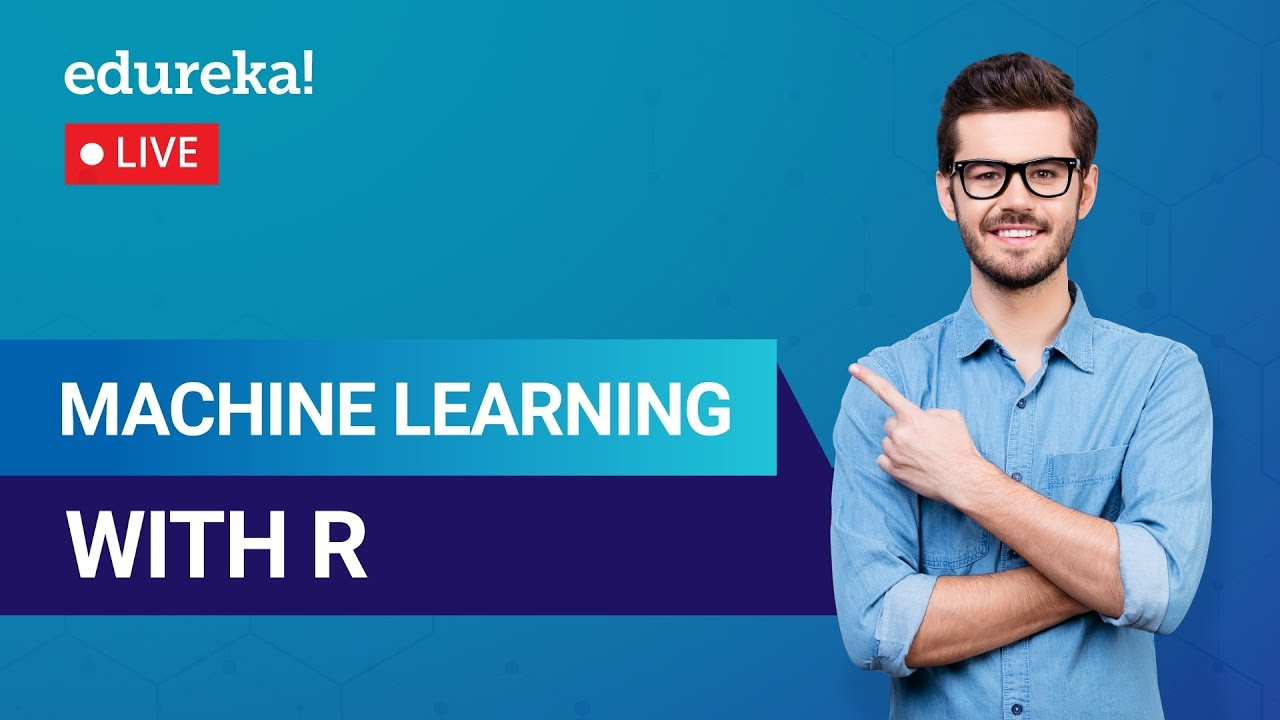# Machine Learning AlgorithmsMachine Learning Python

#### Machine Learning With Python Full Course for Beginners

In this video from Simplilearn learn the basics of machine learning, essential applications of machine learning, and machine learning concepts, and why mathematics, statistics, and linear algebra are crucial. We’ll also learn about regularization, dimensionality reduction, and PCA.AI Solar

#### How AI Could Solve Our Renewable Energy Problem

This video takes a look at how machine learning is building a more renewable electrical grid with solar and wind power, and how it’s starting to impact us.Machine Learning

#### How to Get Started with Machine Learning using Spark MLlib

This Edureka “Machine Learning using Spark MLlib” video will help you to get started with Machine Learning and MLLib.AI Career

#### AI And Machine Learning Full Course Updated for 2022

This Artificial Intelligence and Machine Learning full course from SimpliLearn covers all the topics that you need to know to become a master in the field of AI and Machine Learning.Python

#### Logistic Regression in Python

This Edureka Video on Logistic Regression in Python will give you basic understanding of Logistic Regression Machine Learning Algorithm with examples. Time stamps: 00:00 Introduction 00:31 Agenda 01:13 What is Regression 03:25 Logistic Regression- What and Why 04:10 Why not Linear Regression 05:18 Logistic Regression curve 06:57 Logistic Regression Equation 08:49 Linear vs Logistic Regression […]Machine Learning

#### House Price Prediction using Machine Learning

This Edureka video on predicting home prices will explore core Machine Learning concepts.Machine Learning

#### Naive Bayes Classifier Explained

Here’s a clear explanation of a Naive Bayes Classifier.Machine Learning

#### The Most Important Topic in Machine Learning right now

Karolina Sowinska  discusses the most important topic in Machine Learning right now, namely model explainability. It is one of the hottest discussion points in the data community, because ultimately if we cannot understand how the models arrive at the predictions, it renders them useless in many practical applications.